2018-08-31 12:19:29 atansuozhe 阅读数 182
• ###### MATLAB图像处理

全面系统的学习MATLAB在图像处理中的应用

19898 人正在学习 去看看 魏伟

2019-04-26 23:50:29 zaishuiyifangxym 阅读数 569
• ###### MATLAB图像处理

全面系统的学习MATLAB在图像处理中的应用

19898 人正在学习 去看看 魏伟1 什么是阈值化- threshold()

2 二进制阈值化

3 反二进制阈值化

4 截断阈值化

5 反阈值化为0

6 阈值化为0

7 小结

# 1 什么是阈值化- threshold()

当灰度Gray大于或等于阈值T时，其Y值为255，表示白色。

Python OpenCV中提供了阈值函数 threshold() 实现二值化处理，其函数形式及参数如下图所示：

retval, dst = cv2.threshold(src, thresh, maxval, type)

retval：阈值

dst： 处理结果

src,：原图像

thresh：阈值

maxval：最大值

type：类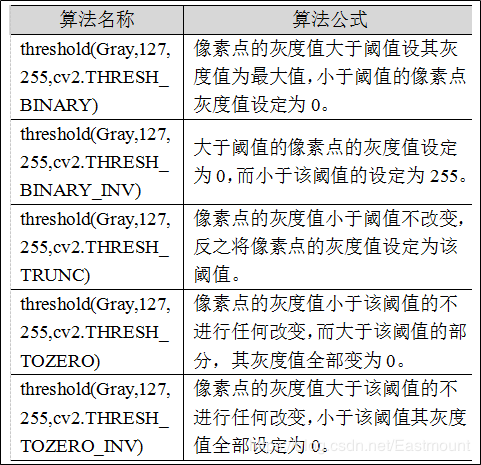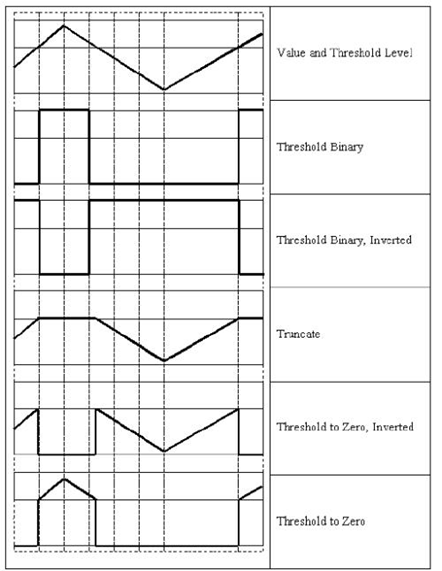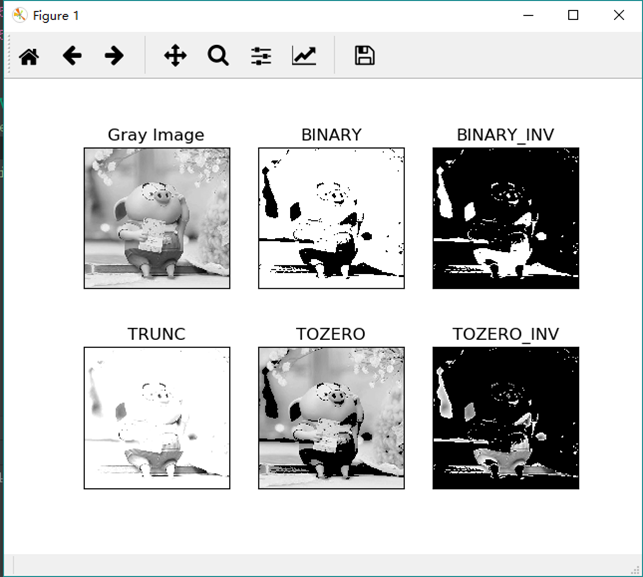# 2 二进制阈值化

(1) 大于等于127的像素点的灰度值设定为最大值（如8位灰度值最大为255）；

(2) 灰度值小于127的像素点的灰度值设定为0 ；

#encoding:utf-8
import cv2
import numpy as np

#读取图片

#灰度图像处理
GrayImage = cv2.cvtColor(src,cv2.COLOR_BGR2GRAY)

#二进制阈值化处理
r, b = cv2.threshold(GrayImage, 127, 255, cv2.THRESH_BINARY)
print (r)

#显示图像
cv2.imshow("src", src)
cv2.imshow("result", b)

#等待显示
cv2.waitKey(0)
cv2.destroyAllWindows()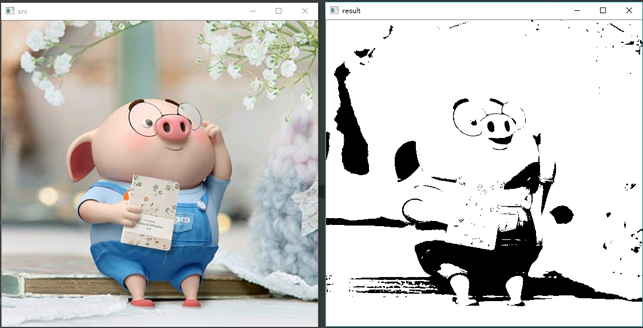# 3 反二进制阈值化

(1) 大于127的像素点的灰度值设定为0（以8位灰度图为例）；

(2) 小于该阈值的灰度值设定为255；

#encoding:utf-8
import cv2
import numpy as np

#读取图片

#灰度图像处理
GrayImage = cv2.cvtColor(src,cv2.COLOR_BGR2GRAY)

#反二进制阈值化处理
r, b = cv2.threshold(GrayImage, 127, 255, cv2.THRESH_BINARY_INV)
print (r)

#显示图像
cv2.imshow("src", src)
cv2.imshow("result", b)

#等待显示
cv2.waitKey(0)
cv2.destroyAllWindows()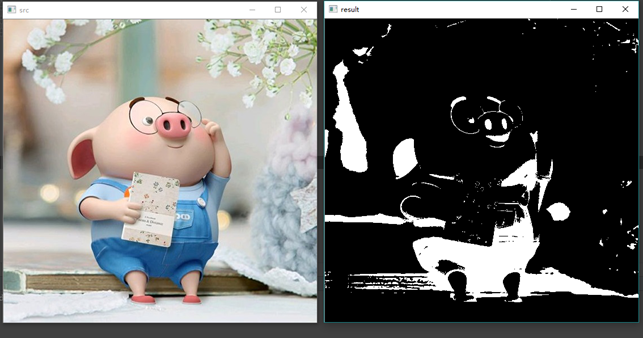# 4 截断阈值化

(1) 大于等于127的像素点的灰度值设定为该阈值127；

(2) 小于该阈值的灰度值不改变；

#encoding:utf-8
import cv2
import numpy as np

#读取图片

#灰度图像处理
GrayImage = cv2.cvtColor(src,cv2.COLOR_BGR2GRAY)

#截断阈值化处理
r, b = cv2.threshold(GrayImage, 127, 255, cv2.THRESH_TRUNC)
print (r)

#显示图像
cv2.imshow("src", src)
cv2.imshow("result", b)

#等待显示
cv2.waitKey(0)
cv2.destroyAllWindows()# 5 反阈值化为0

(1) 大于等于阈值127的像素点变为0 ；

(2) 小于该阈值的像素点值保持不变；

#encoding:utf-8
import cv2
import numpy as np

#读取图片

#灰度图像处理
GrayImage = cv2.cvtColor(src,cv2.COLOR_BGR2GRAY)

#反阈值化为0处理
r, b = cv2.threshold(GrayImage, 127, 255, cv2.THRESH_TOZERO_INV)
print (r)

#显示图像
cv2.imshow("src", src)
cv2.imshow("result", b)

#等待显示
cv2.waitKey(0)
cv2.destroyAllWindows()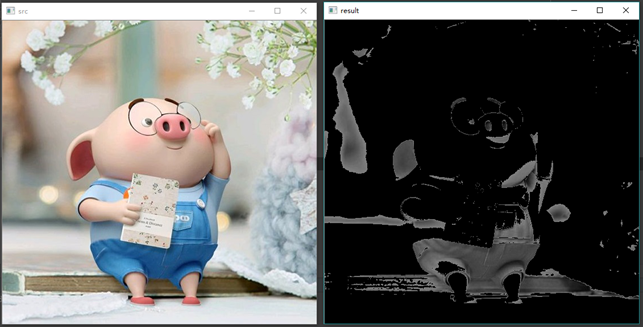# 6 阈值化为0

(1) 大于等于阈值127的像素点，值保持不变；

(2) 小于该阈值的像素点值设置为0 ；

#encoding:utf-8
import cv2
import numpy as np

#读取图片

#灰度图像处理
GrayImage = cv2.cvtColor(src,cv2.COLOR_BGR2GRAY)

#阈值化为0处理
r, b = cv2.threshold(GrayImage, 127, 255, cv2.THRESH_TOZERO)
print (r)

#显示图像
cv2.imshow("src", src)
cv2.imshow("result", b)

#等待显示
cv2.waitKey(0)
cv2.destroyAllWindows()# 7 小结

#encoding:utf-8
import cv2
import numpy as np
import matplotlib.pyplot as plt

#读取图像
lenna_img = cv2.cvtColor(img,cv2.COLOR_BGR2RGB)
GrayImage=cv2.cvtColor(img,cv2.COLOR_BGR2GRAY)

#阈值化处理
ret,thresh1=cv2.threshold(GrayImage,127,255,cv2.THRESH_BINARY)
ret,thresh2=cv2.threshold(GrayImage,127,255,cv2.THRESH_BINARY_INV)
ret,thresh3=cv2.threshold(GrayImage,127,255,cv2.THRESH_TRUNC)
ret,thresh4=cv2.threshold(GrayImage,127,255,cv2.THRESH_TOZERO)
ret,thresh5=cv2.threshold(GrayImage,127,255,cv2.THRESH_TOZERO_INV)

#显示结果
titles = ['Gray Image','BINARY','BINARY_INV','TRUNC','TOZERO','TOZERO_INV']
images = [GrayImage, thresh1, thresh2, thresh3, thresh4, thresh5]
for i in range(6):
plt.subplot(2,3,i+1),plt.imshow(images[i],'gray')
plt.title(titles[i])
plt.xticks([]),plt.yticks([])
plt.show()# 参考资料

2018-01-24 18:57:06 baidu_33939056 阅读数 5714
• ###### MATLAB图像处理

全面系统的学习MATLAB在图像处理中的应用

19898 人正在学习 去看看 魏伟

1，binary dilation，用来去除一些灰度值小的噪声。在具体应用中如何选取核需要尝试
scipy 中有现成的包可以调用 链接

2，Otsu’s method，用来对图像进行二值化，可以用来区分前景和背景。利用opencv 代码如下：

cv2.threshold(gray_image, 0, 255, cv2.THRESH_OTSU)

3,flood fill algorithm:文档,l实例程序

2017-04-01 13:46:29 hqxdamowang 阅读数 2235
• ###### MATLAB图像处理

全面系统的学习MATLAB在图像处理中的应用

19898 人正在学习 去看看 魏伟

（二）屋顶状边缘：边缘处于灰度值有小到大再到小变化的转折点处。

Canny算子：Canny 的目标是找到一个最优的边缘检测算法，最优边缘检测的含义是： 好的检测- 算法能够尽可能多地标识出图像中的实际边缘。 好的定位- 标识出的边缘要尽可能与实际图像中的实际边缘尽可能接近。 最小响应- 图像中的边缘只能标识一次，并且可能存在的图像噪声不应标识为边缘。 为了满足这些要求 Canny 使用了变分法，这是一种寻找满足特定功能的函数的方法。最优检测使用四个指数函数项的和表示，但是它非常近似于高斯函数的一阶导数。

Harris角点：人眼对角点的识别通常是在一个局部的小区域或小窗口完成的。如果在各个方向上移动这个特征的小窗口，窗口内区域的灰度发生了较大的变化，那么就认为在窗口内遇到了角点。如果这个特定的窗口在图像各个方向上移动时，窗口内图像的灰度没有发生变化，那么窗口内就不存在角点；如果窗口在某一个方向移动时，窗口内图像的灰度发生了较大的变化，而在另一些方向上没有发生变化，那么，窗口内的图像可能就是一条直线的线段。

1.准确性：即使很细小的角点，算法也能检测到；

2.定位性：检测到的角点应尽可能地接近它们在图像中的真实位置；

3稳定性：对相同场景拍摄的多幅图片，每一个角点的位置都不应该移动；

4.实时性：角点检测算法的计算量越小、运行的速度越快越好；

5.鲁棒性：对噪声具有抗干燥性。

surf特征提取：计算机视觉中，引入尺度不变的特征，主要的思想是每个检测到的特征点都伴随着对应的尺寸因子。当我们想匹配不同图像时，经常会遇到图像尺度不同的问题，不同图像中特征点的距离变得不同，物体变成不同的尺寸，如果我们通过修正特征点的大小，就会造成强度不匹配。为了解决这个问题，提出一个尺度不变的SURF特征检测，在计算特征点的时候把尺度因素加入之中。SURF与SIFT算法相似，SIFT算法比较稳定，检测特征点更多，但是复杂度较高，而SURF要运算简单，效率高，运算时间短一点。

2018-01-04 20:20:28 coming_is_winter 阅读数 571
• ###### MATLAB图像处理

全面系统的学习MATLAB在图像处理中的应用

19898 人正在学习 去看看 魏伟

图像处理之前景检测（一）之单高斯模型

运动前景对象检测一直是国内外视觉监控领域研究的难点和热点之一，其目的是从序列图像中将变化区域从背景图像中提取出来，运动前景对象的有效检测对于对象跟踪、目标分类、行为理解等后期处理至关重要，那么区分前景对象，非常关键的一个问题是确定一个非常合适的背景，背景从象素的角度来理解，每一个象素就是有可能是前景点，也有可能是背景点，那么我们就要防止背景中误进入原属于前景点的对象，目前有几种常用的方法，但分别有利弊。

具体类型见（参考及推荐文献）：

这里按照顺序，将感兴趣的有关算法进行梳理。首先是单高斯模型。

单高斯模型建模比较简单，利用没有运动目标的图像将整个窗口进行高斯建模，对比后续对应点上的像素数据，符合高斯模型，持续为背景，超出某个阈值，则认为为前景。

具体原理参考：

沈春旭    运动目标检测_单高斯背景建模

这里主要将  zhengtu009  的  单高斯背景建模原理及代码实现  中的C接口代码进行升级为C++接口。环境为VS2015+opencv3.2 contrib版。

#include<iostream>
#include<opencv.hpp>
using namespace std;
using namespace cv;

int main(int argc, char* argv[])
{
//新建窗口
namedWindow("origin", WINDOW_AUTOSIZE);
namedWindow("background", WINDOW_AUTOSIZE);
namedWindow("foreground", WINDOW_AUTOSIZE);

double alpha = 0.05;    //背景建模alpha值
double std_init = 20;    //初始标准差
double var_init = std_init * std_init;    //初始方差
double lamda = 2.5 * 1.2;    //背景更新参数

//视频文件
VideoCapture capture("E://素材//3.mp4");

Mat frame;        //原始图像

Scalar pixel = { 0 };        //像素原始值
Scalar pixel_u = { 0 };        //像素期望
Scalar pixel_d = { 0 };        //像素前景
Scalar pixel_var = { 0 };    //像素方差
Scalar pixel_std = { 0 };    //像素标准差

capture >> frame;
Mat frame_u(frame.rows,frame.cols,  CV_8UC3);
Mat frame_d(frame.rows,frame.cols , CV_8UC3);
Mat frame_var(frame.rows,frame.cols,  CV_8UC3);
Mat frame_std(frame.rows,frame.cols,  CV_8UC3);

for (int y = 0; y < frame.rows; ++y)
{
for (int x = 0; x < frame.cols; ++x)
{
pixel =frame.at<Vec3b>(y,x);
pixel_u.val = pixel.val;
pixel_u.val = pixel.val;
pixel_u.val = pixel.val;

pixel_d.val = 0;
pixel_d.val = 0;
pixel_d.val = 0;

pixel_std.val = std_init;
pixel_std.val = std_init;
pixel_std.val = std_init;

pixel_var.val = var_init;
pixel_var.val = var_init;
pixel_var.val = var_init;

//cvSet2D(frame_u, y, x, pixel_u);
frame_u.at<Vec3b>(y, x) = pixel_u;
frame_u.at<Vec3b>(y, x) = pixel_u;
frame_u.at<Vec3b>(y, x) = pixel_u;
frame_d.at<Vec3b>(y, x) = pixel_d;
frame_d.at<Vec3b>(y, x) = pixel_d;
frame_d.at<Vec3b>(y, x) = pixel_d;
frame_var.at<Vec3b>(y, x) = pixel_var;
frame_var.at<Vec3b>(y, x) = pixel_var;
frame_var.at<Vec3b>(y, x) = pixel_var;
frame_std.at<Vec3b>(y, x) = pixel_std;
frame_std.at<Vec3b>(y, x) = pixel_std;
frame_std.at<Vec3b>(y, x) = pixel_std;
}
}
while (1)        //按ESC键退出, 帧率33ms
{
capture>>frame ;
//视频结束退出
if (frame.empty()==1)
{
break;
}
//单高斯背景更新
for (int y = 0; y < frame.rows; ++y)
{
for (int x = 0; x < frame.cols; ++x)
{

pixel = frame.at<Vec3b>(y, x);

pixel_u = frame_u.at<Vec3b>(y, x);

pixel_d = frame_d.at<Vec3b>(y, x);

pixel_std = frame_std.at<Vec3b>(y, x);

pixel_var = frame_var.at<Vec3b>(y, x);

//|I-u| < lamda*std 时认为是背景, 进行更新
if (fabs(pixel.val - pixel_u.val) < lamda * pixel_std.val &&
fabs(pixel.val - pixel_u.val) < lamda * pixel_std.val &&
fabs(pixel.val - pixel_u.val) < lamda * pixel_std.val)
{
//更新期望 u = (1-alpha)*u + alpha*I
pixel_u.val = (1 - alpha) * pixel_u.val + alpha * pixel.val;
pixel_u.val = (1 - alpha) * pixel_u.val + alpha * pixel.val;
pixel_u.val = (1 - alpha) * pixel_u.val + alpha * pixel.val;

//更新方差 var = (1-alpha)*var + alpha*(I-u)^2
pixel_var.val = (1 - alpha) * pixel_var.val +
(pixel.val - pixel_u.val) * (pixel.val - pixel_u.val);
pixel_var.val = (1 - alpha) * pixel_var.val +
(pixel.val - pixel_u.val) * (pixel.val - pixel_u.val);
pixel_var.val = (1 - alpha) * pixel_var.val +
(pixel.val - pixel_u.val) * (pixel.val - pixel_u.val);

//更新标准差
pixel_std.val = sqrt(pixel_var.val);
pixel_std.val = sqrt(pixel_var.val);
pixel_std.val = sqrt(pixel_var.val);

//写入矩阵
//cvSet2D(frame_u, y, x, pixel_u);
frame_u.at<Vec3b>(y, x) = pixel_u;
frame_u.at<Vec3b>(y, x) = pixel_u;
frame_u.at<Vec3b>(y, x) = pixel_u;
//cvSet2D(frame_var, y, x, pixel_var);
//cvSet2D(frame_std, y, x, pixel_std);
frame_var.at<Vec3b>(y, x) = pixel_var;
frame_var.at<Vec3b>(y, x) = pixel_var;
frame_var.at<Vec3b>(y, x) = pixel_var;
frame_std.at<Vec3b>(y, x) = pixel_std;
frame_std.at<Vec3b>(y, x) = pixel_std;
frame_std.at<Vec3b>(y, x) = pixel_std;
}
else
{
pixel_d.val = pixel.val - pixel_u.val;
pixel_d.val = pixel.val - pixel_u.val;
pixel_d.val = pixel.val - pixel_u.val;
//cvSet2D(frame_d, y, x, pixel_d);
frame_d.at<Vec3b>(y, x) = pixel_d;
frame_d.at<Vec3b>(y, x) = pixel_d;
frame_d.at<Vec3b>(y, x) = pixel_d;
}
}
}
imshow("origin", frame);
imshow("background", frame_u);
imshow("foreground", frame_d);
waitKey(20);
}

return 0;
}
代码只是对于函数进行升级，但实现结果较老版些许不同，不知是版本更新问题，还是升级代码存在未知错误，大家辩证看待。

原代码结果：升级代码结果：结束。

视频素材下载地址：行人检测